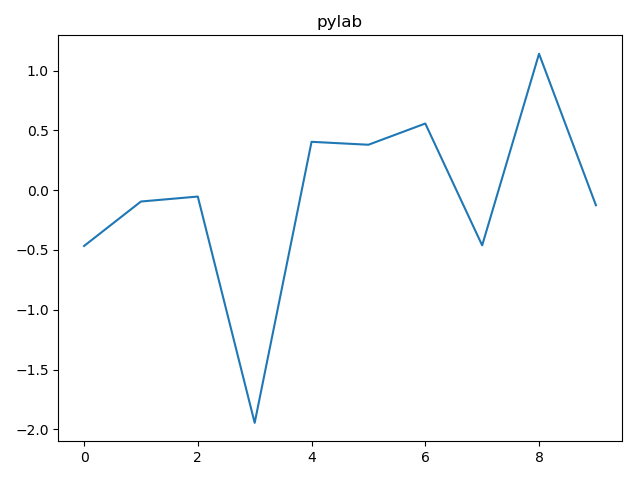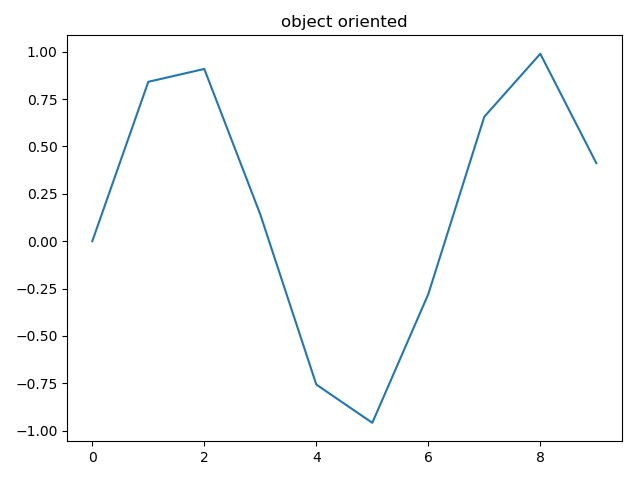# Matplotlib可视化（十）–面向对象绘图 VS matlabstyle587-王同学

,

## 三种方式简介： 1.pyplot：经典高层封装，到目前为止，我们所用的都是pyplot 2.pylab：将matlab和numpy合并的模块，模拟matlab的编程环境 3.面对对象的方式：matplotlib的精髓，更基础和底层的方式

• pylab

from pylab import *
x = arange(10)
y = randn(len(x))
plot(x, y)
title('pylab')
show()• 面向对象
import matplotlib.pyplot as plt
import numpy as np
x = np.arange(10)
# y = np.random.randn(len(x))
y = np.sin(x)
fig = plt.figure()#生成一张画布对象
l,=plt.plot(x, y)
t = ax.set_title('object oriented')
plt.show()### 评论 抢沙发### 觉得文章有用就打赏一下文章作者

#### 支付宝扫一扫打赏#### 微信扫一扫打赏Vieu3.3主题Q Q 登 录